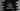# C++ program to find the larger string# Find out the larger string in C++ :

To find out the larger string, you need to find out its length. In C++, we can find out the length of a string using the strlen method. In this tutorial, we will learn how to find out the length of the string, and how to find the larger string in C++.

## strlen() in C++ :

strlen() is used to find out the length of a string. It is defined in cstring header file. cstring is used for c-style null-terminated byte string. It includes a lot of useful string methods and strlen() is one among them.

This method is defined as below :

``````size_t strlen( const char* str );
``````

It takes one C style string as its argument and returns its size.

## Example to find the largest string in C++ :

``````#include <iostream>
#include <cstring>
using namespace std;

int main()
{
string str1, str2;

cout << "Enter the first string : " << endl;
getline(cin, str1);
cout << "Enter the second string : " << endl;
getline(cin, str2);

if (strlen(str1.c_str()) == strlen(str2.c_str()))
{
cout << "Both strings are equal" << endl; } else if (strlen(str1.c_str()) > strlen(str2.c_str()))
{
cout << "The first string is larger than the second string" << endl;
}
else
{
cout << "The first string is smaller than the second string" << endl;
}
return 0;
}
``````

In this program, we are asking the user to enter the length of the first and the second string. It uses the strlen() method to find out the length of both strings. We are using c_str() method to covert the string to c_str or c type string. With a simple if-else if-else statement, we can compare both strings.

### Example outputs :

``````Enter the first string :
hello
Enter the second string :
world
Both strings are equal

Enter the first string :
one
Enter the second string :
an
The first string is larger than the second string

Enter the first string :
hello
Enter the second string :
hello world
The first string is smaller than the second string
``````